# TweenCurves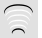Curve

Tween CurvesHistory enabled

The TweenCurves command creates curves between two open or closed input curves.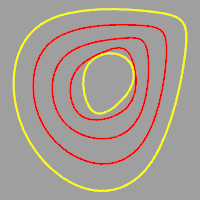#### Steps

1. Select the first curve.
2. Select the second curve.
3. Adjust the curve if necessary.
Click a curve endpoint to change direction. A preview of the curves changes each time the endpoint is clicked.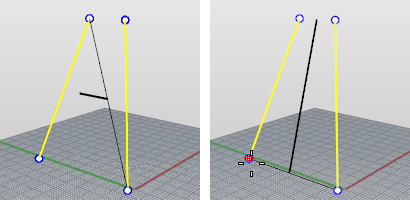Before changing curve endpoint (left) and after clicking the left curve endpoint (right).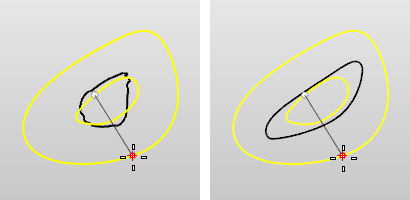Before changing curve endpoint (left) and after clicking the left curve endpoint (right).
Command-line options
##### Number

Specifies the number of curves to create between the two input curves.

##### CurrrentLayer

Places new curves on the current layer.

##### StartCrv

Places new curves on the same layer as the first curve selected.

##### EndCrv

Places new curves on the same layer as the second curve selected.

##### MatchMethod

Specifies the method for refining the output curves.

##### None

Uses the control points of the curves for matching. So the first control point of first curve is matched to first control point of the second curve.

##### Refit

Refits the output curves like using the FitCrv command. Both the input curve and the output curve will have the same structure. The resulting curves are usually more complex than input unless input curves are compatible.

##### SamplePoints

Input curves are divided to the specified number of points on the curve, corresponding points define new points that output curves go through.

If you are making one tween curve, the command essentially does the following:

Divides the two curves into an equal number of points, finds the midpoint between the corresponding points on the curves. and interpolates the tween curve through those points.

##### SampleNumber

The number of sample points to use.

##### Flip

Changes the direction of one the curves.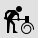##### Rebuild

Reconstruct curves, surfaces, and extrusion objects to a specified degree and control point number.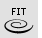##### FitCrv

Make a non-rational NURBS curve of a specified degree that matches the input curve to within the specified tolerance.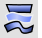##### TweenSurfaces

Create intermediate surfaces between two input surfaces.

Rhinoceros 7 © 2010-2023 Robert McNeel & Associates. 03-May-2023